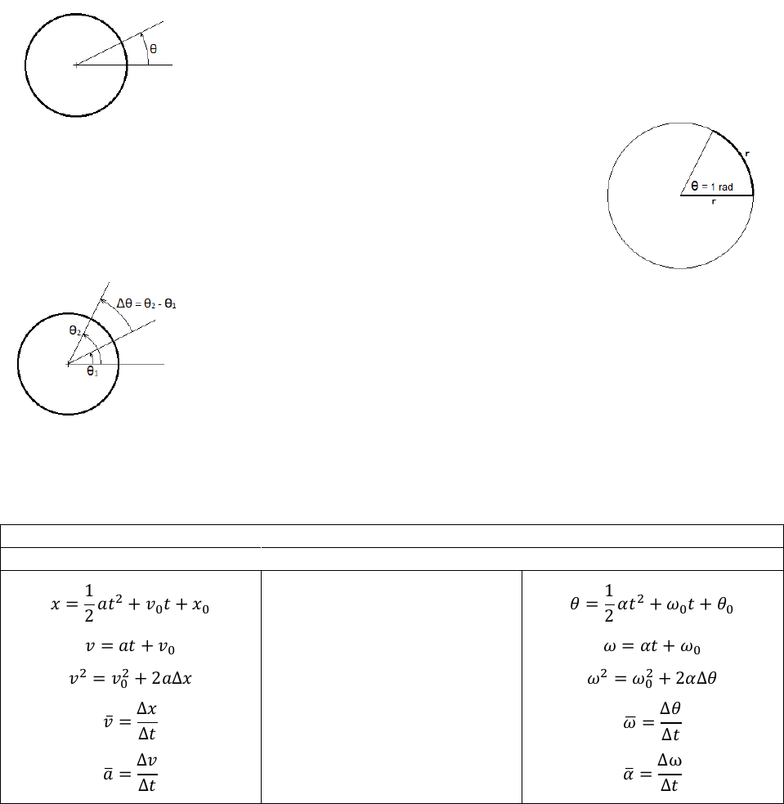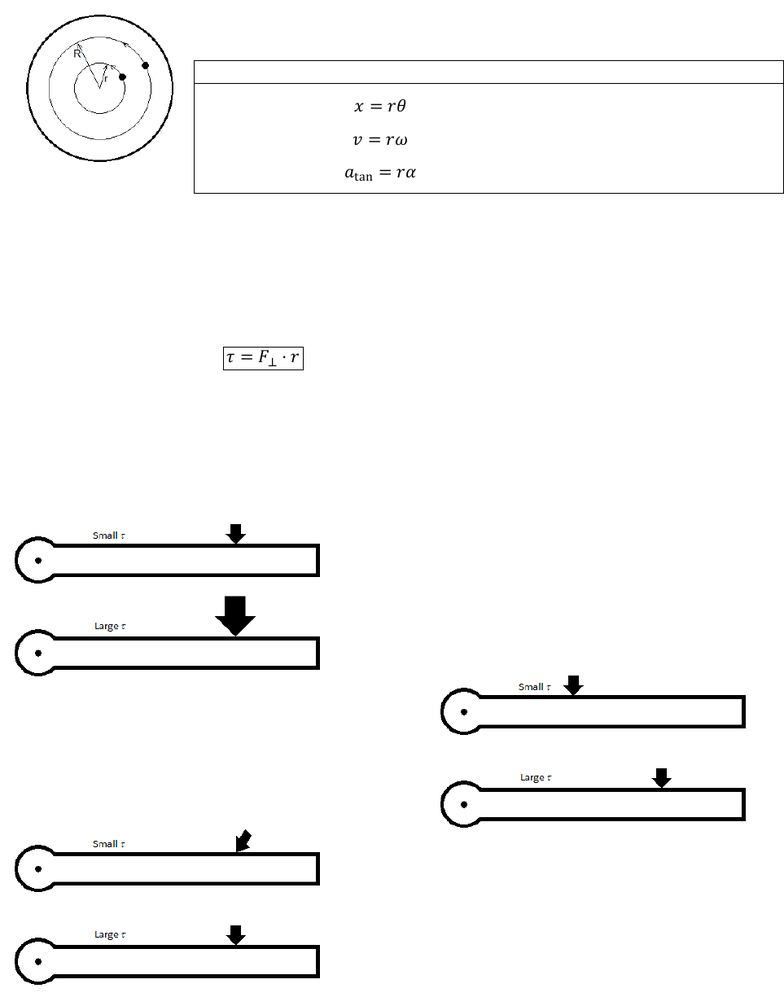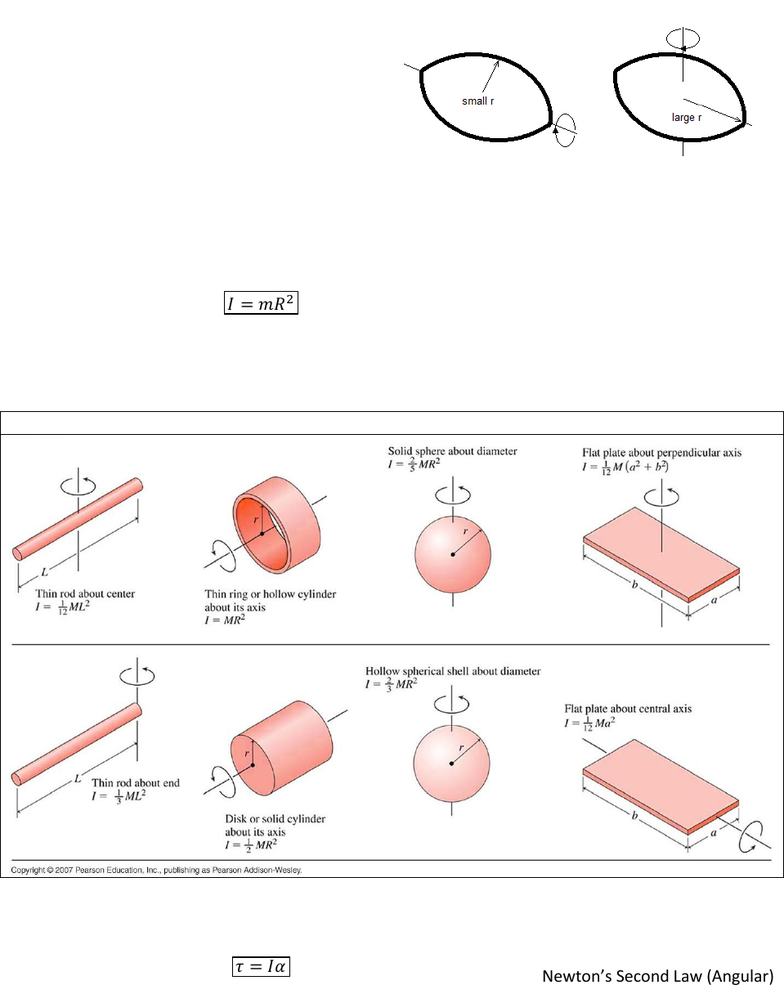# PHYS 2108 Lecture Notes - Lecture 1: Angular Acceleration, Burma Plate, Radian

71 views9 pages
School
Department
Course
Professor

For unlimited access to Study Guides, a Grade+ subscription is required.7-1
LAB 7:
Rotational Motion
The most basic field of study in physics is kinematics: position, velocity and acceleration. It’s the first
topic and contains the most basic (and often used) equations in physics.
Kinematics doesn’t always have to happen in a straight line. Rather than
travelling across some distance x, it’s possible to consider spinning through
some rotational “distance.” We usually choose to represent the angular
displacement as the Greek letter theta, .
We have a special unit of measure for angles that you may have seen in your
calculator called radians. Radians are a more natural unit in the universe
than degrees because radians are the result of ratios. Because of that they’re
one of the very few units that you can stick in when you need them or
remove when you don’t. If you lay a string as long as a circle’s radius along
the edge of the circle, the angle it sweeps out is equal to one radian.
If it’s possible to travel through an angle, it’s possible to do it over some time
at some kind of “speed.” If we were to rotate the pictured disk from θ1 to θ2 in
some time Δt, it seems we have invented a fairly logical equation for the
angular speed or angular velocity of a rotating system. We use a lower case
omega ω to represent it and the units are in rad/s or more familiarly,
Revolutions Per Minute (RPM). Similarly, if we change the angular speed, we
can make a reasonable argument for an angular acceleration α.
It turns out that every formula we had in kinematics for straight-line motion has an angular analogue.
Don’t let angular problems intimidate you! You’ve done similar problems before with different symbols!
Eqn. Table 7-1: Translational and Rotational Kinematics
Translational (Linear) Equations
Rotational (Angular) Equations
displacement

velocity

the “timeless” equation
average velocity
average acceleration

Unlock document

This preview shows pages 1-3 of the document.
Unlock all 9 pages and 3 million more documents.

Already have an account? Log in7-2
If two dots are painted on a spinning disk, they must have the same angular speed (because they’re
connected to the disk). Do they have the same linear speed? In one revolution, the outer dot travels
2πR while the inner one travels only 2πr. Because the outer dot has to cross a greater distance in the
same amount of time, it must do it more quickly. Linear (or “tangential”)
quantities and angular ones can be converted as follows:
Eqn. Table 7-2: Linear-Angular Conversions


 
Newton’s Second Law (Angular)
Try to push a door open near the knob, then again near the hinges. Which one is easier?
When thinking about rotation, sheer force alone is not as important as where it’s applied. Forces
applied close to the rotation axis or pivot point are less effective than those applied much further away.
This combination of force and its point of application is called torque in units of meter-Newtons (m-N).
Eqn. 7-3:
Definition of Torque
In the formula, (tau) represents the torque, is the radius between the rotation axis and the location
of the force, and is the component of the force perpendicular to the radius. Any components
parallel to the radius are trying to pull the door off its hinges, not trying to rotate it.
There are apparently three ways to affect the torque on a rotating system:
Change the amount of force. Pushing harder generates
greater torque.
Change the distance from the pivot point. Pushing
farther away generates greater torque.
Change the angle in which the force is applied. Pushing
more directly generates greater torque. Components
parallel to the radius do not contribute to rotation.
Unlock document

This preview shows pages 1-3 of the document.
Unlock all 9 pages and 3 million more documents.

Already have an account? Log in7-3
Try to spin a football on its point (like one thrown
in a good spiral, left diagram), then spin it again on
its equator (right diagram). Which is easier?
Much like the case with force, when trying to
angularly accelerate something not only is its mass
important, but also where the mass is distributed.
Because more of the football’s mass is closer to
the rotation axis in the leftward case, it has a
smaller rotational mass” and the same torque can
better rotate it than it can in the rightward case.
This rotational mass” is called the moment of inertia and is denoted by a capital . For any point-like
mass some distance R from the axis of rotation:
Eqn. 7-4:
Moment of Inertia (Point Mass)
Most real-life objects aren’t point-masses. By using calculus to add up mR2 for every atom in an
extended body, we find moments of inertia for the following shapes.
Eqn. Table 7-5: Moments of Inertia (Extended Bodies)
Knowing that torque acts on a moment of inertia to produce rotational acceleration, we can invent an
angular version of Newton’s Second Law.

Eqn. 7-6:
Newton’s Second Law (Angular)
Unlock document

This preview shows pages 1-3 of the document.
Unlock all 9 pages and 3 million more documents.

Already have an account? Log in

# Get access

Grade+
\$10 USD/m
Billed \$120 USD annually
Homework Help
Class Notes
Textbook Notes
40 Verified Answers
Study Guides
1 Booster Class